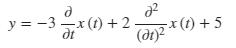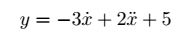# Revision history [back]

### Pretty Print in Newton Notation?

Is there any way to get the pretty printer to produce Newton's notation? - ie. a single dot centred over the variable for first derivative with respect to time, 2 dots for second derivative etc.

### Pretty Print in Newton Notation?

Is there any way to get the pretty printer to produce Newton's notation? - ie. a single dot centred over the variable for first derivative with respect to time, 2 dots for second derivative etc.

Example:

t, y = var('t, y')


x = function('x')(t) pretty_print(y == 2*diff(diff(x,t),t) - 3 * diff(x,t) + 5)

### Pretty Print in Newton Notation?

Is there any way to get the pretty printer to produce Newton's notation? - ie. a single dot centred over the variable for first derivative with respect to time, 2 dots for second derivative etc.

Example:

t, y = var('t, y')


x = function('x')(t) pretty_print(y == 2*diff(diff(x,t),t) - 3 * diff(x,t) + 5)5)

gives:but I'd like to see:4 None

### Pretty Print in Newton Notation?

Is there any way to get the pretty printer to produce Newton's notation? - ie. a single dot centred over the variable for first derivative with respect to time, 2 dots for second derivative etc.

Example:

t, y = var('t, y')
x = function('x')(t)
pretty_print(y == 2*diff(diff(x,t),t) - 3 * diff(x,t) + 5)


gives:but I'd like to see: